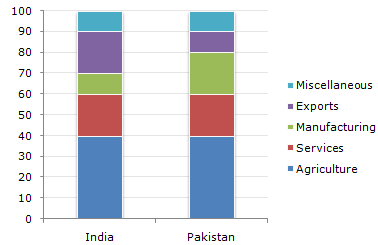# Data Interpretation - Bar Charts

The following bar chart shows the composition of the GDP two countries (India and Pakistan).

Composition of GDP of Two Countries1.

If the total GDP of Pakistan is Rs. 10,000 crore, then a GDP accounted for by Manufacturing is ?

 A. Rs.200 crore B. Rs.600 crore C. Rs.2,000 crore D. Rs.6,000 crore

Explanation:

20% of 10000 = 2000

2.

What fraction of India's GDP is accounted for by Services ?

 A. (6/33)th B. (1/5)th C. (2/3)rd D. None of these

Explanation:

Service accounts for 20%, i.e., (1/5)th of the GDP of India.

3.

If the total GDP of India is Rs.30,000 crores, then the GDP accounted for by Agriculture, Services and Miscellaneous is ?

 A. Rs.18,500 crore B. Rs.18,000 crore C. Rs.21,000 crore D. Rs.15,000 crore

Explanation:

(40 + 20 + 10)% of 30,000 = Rs.21,000 crore.

4.

Which country accounts for higher earning out of Services and Miscellaneous together ?

 A. India B. Pakistan C. Both spend equal amounts D. Cannot be determined

Explanation:

Although the percentage on Services and Miscellaneous put together is equal for both the countries, we cannot comment on this since we have no data about the respective GDP's.

5.

If the total GDP is the same for both the countries, then what percentage is Pakistan's income through agriculture over India's income through Services ?

 A. 100 % B. 200 % C. 133.33 % D. None of these